﻿ 基于三维Copula函数的组合洪水研究 Combined Flood Research Based on Three-Dimensional Copula Function

Journal of Water Resources Research
Vol. 09  No. 01 ( 2020 ), Article ID: 33670 , 9 pages
10.12677/JWRR.2020.91004

Combined Flood Research Based on Three-Dimensional Copula Function

Xijin Liang, Liping Wang, Yanke Zhang*, Jiaxin Zhang, Kaihua Ye

Renewable Energy School, North China Electric Power University, Beijing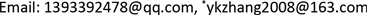Received: Dec. 7th, 2019; accepted: Dec. 20th, 2019; published: Dec. 27th, 2019ABSTRACT

When a river has multiple tributaries, the occurrence of mainstream floods and tributary floods is the main factor threatening the safety of downstream flood control points. The risk assessment of floods encountering is important for downstream flood control safety. Based on the Copula function which is easy to construct multiple edge distributions into multi-dimensional joint distribution, combining with the frequency composition method, the expression of n-dimensional combined flood distribution function is derived and the corresponding flood encounter risk estimation model is established. Taking the floods encountering of the Yalong River, the Xianshui River, and the Qingda River as an example, and using the two-parameter three-dimensional Copula function to estimate the downstream flood risk, the downstream combined flood distribution function and flood control risk rate were calculated. The results show that the model can quantitatively analyze the randomness of floods encountering and provide a certain reference for downstream flood control.

Keywords:Copula Function, Frequency Composition Method, Combined Flood, Flood Risk Rate of Flood Control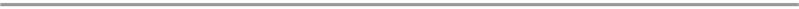1. 引言

Copula函数能够通过变量的边缘分布和相关性结构两部分来构造多维联合分布，形式灵活多样，是构建联合分布的一种非常有效的方法，近些年在水文分析计算领域中得到了广泛应用。熊立华等  采用Copula函数构造了两站年最大洪水联合分布函数，并且对经验概率和理论概率进行了拟合。李天元等  通过双参数Copula函数构造了两江洪峰之间的联合分布函数，估计了洪峰遭遇风险的条件概率。刘章君等  根据Copula函数对设计洪水地区组成进行区间估计，计算出地区组成的点估计值，并对估计的不确定性进行评价。

2. Copula函数

Copula函数由Sklar在1959年提出，是定义在[0,1]上均匀分布的联合分布函数。其中Archimedean Copula函数结构简单，适应性强，在水文多变量联合分布中的应用较为广泛。n维Archimedean Copula函数表达式如下：

$C\left(u,\phi \right)=\phi \left\{{\phi }^{-1}\left({u}_{1}\right)+{\phi }^{-1}\left({u}_{2}\right)+\cdots +{\phi }^{-1}\left({u}_{n}\right)\right\},u\in \left[0,1\right]$ (1)

$C\left({u}_{1},{u}_{2},{u}_{3}\right)={\phi }_{1}\left({\phi }_{1}^{-1}\left({u}_{1}\right)+{\phi }_{1}^{-1}{\phi }_{2}\left({\phi }_{2}^{-1}\left({u}_{2}\right)+{\phi }_{2}^{-1}\left({u}_{3}\right)\right)\right)$ (2)

$C\left({u}_{1},{u}_{2},{u}_{3};{\theta }_{1},{\theta }_{2}\right)=\mathrm{exp}\left\{-{\left({\left[{\left(-\mathrm{log}{u}_{3}\right)}^{{\theta }_{2}}+{\left(-\mathrm{log}{u}_{2}\right)}^{{\theta }_{2}}\right]}^{{\theta }_{1}/{\theta }_{2}}+{\left(-\mathrm{log}{u}_{1}\right)}^{{\theta }_{1}}\right)}^{1/{\theta }_{1}}\right\}$ (3)

$C\left({u}_{1},{u}_{2},{u}_{3};{\theta }_{1},{\theta }_{2}\right)={\left[{\left({u}_{3}^{-{\theta }_{2}}+{u}_{2}^{-{\theta }_{2}}-1\right)}^{{\theta }_{1}/{\theta }_{2}}+{u}_{1}^{-{\theta }_{1}}-1\right]}^{-1/{\theta }_{1}}$ (4)

$C\left({u}_{1},{u}_{2},{u}_{3};{\theta }_{1},{\theta }_{2}\right)=-{\theta }_{1}^{-1}\mathrm{log}\left\{1-{c}_{1}^{-1}\left(1-{\left[1-{c}_{2}^{-1}\left(1-{\text{e}}^{-{\theta }_{2}{u}_{3}}\right)\left(1-{\text{e}}^{-{\theta }_{2}{u}_{2}}\right)\right]}^{{\theta }_{1}/{\theta }_{2}}\right)\left(1-{\text{e}}^{-{\theta }_{1}{u}_{1}}\right)\right\}$ (5)

3. 基于Copula函数的洪水遭遇风险估计模型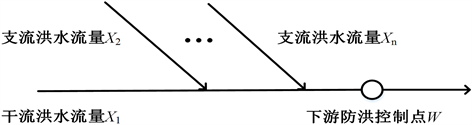Figure 1. Flood encounter combination diagram

$P\left(w\ge {w}_{0}\right)=1-\int \cdots {\int }_{D}h\left({x}_{1},{x}_{2},\cdots ,{x}_{n}\right)\text{d}{x}_{1}\text{d}{x}_{2}\cdots \text{d}{x}_{n}$ (6)

$H\left({x}_{1},{x}_{2},\cdots ,{x}_{n}\right)=C\left[F\left({x}_{1}\right),F\left({x}_{2}\right),\cdots ,F\left({x}_{n}\right)\right]$ (7)

$h\left({x}_{1},{x}_{2},\cdots ,{x}_{n}\right)=\frac{{\partial }^{n}H\left({x}_{1},{x}_{2},\cdots ,{x}_{n}\right)}{\partial {x}_{1}\partial {x}_{2}\cdots \partial {x}_{n}}=\frac{{\partial }^{n}C\left({u}_{1},{u}_{2},\cdots ,{u}_{n}\right)}{\partial {x}_{1}\partial {x}_{2}\cdots \partial {x}_{n}}$ (8)

$\frac{{\partial }^{n}C\left({u}_{1},{u}_{2},\cdots ,{u}_{n}\right)}{\partial {x}_{1}\partial {x}_{2}\cdots \partial {x}_{n}}=\frac{{\partial }^{n}C\left({u}_{1},{u}_{2},\cdots ,{u}_{n}\right)}{\partial {u}_{1}\partial {u}_{2}\cdots \partial {u}_{n}}\cdot \frac{\partial {u}_{1}}{\partial {x}_{1}}\cdot \frac{\partial {u}_{2}}{\partial {x}_{2}}\cdots \frac{\partial {u}_{n}}{\partial {x}_{n}}=c\left({u}_{1},{u}_{2},\cdots ,{u}_{n}\right)\cdot {f}_{{x}_{1}}\left({x}_{1}\right)\cdot {f}_{{x}_{2}}\left({x}_{2}\right)\cdots {f}_{{x}_{n}}\left({x}_{n}\right)$ (9)

$P\left(w\ge {w}_{0}\right)=1-\int \cdots {\int }_{D}c\left({u}_{1},{u}_{2},\cdots ,{u}_{n}\right)\cdot {f}_{{x}_{1}}\left({x}_{1}\right)\cdot {f}_{{x}_{2}}\left({x}_{2}\right)\cdots {f}_{{x}_{n}}\left({x}_{n}\right)\text{d}{x}_{1}\text{d}{x}_{2}\cdots \text{d}{x}_{n}$ (10)

①确定边缘分布，即洪水流量的分布函数。

②在分析边缘分布相关性的基础上，选择合适的Copula函数。

③对Copula函数密度函数以及边缘分布密度函数的乘积求n重积分。

4. 实例分析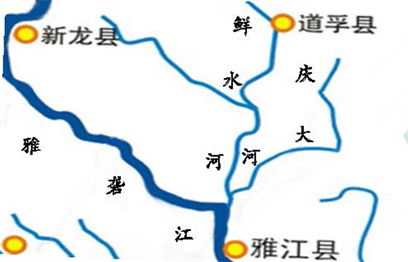Figure 2. Yalong river basin map

4.1. 边缘分布的确定

P-III分布曲线在水文变量频率分析中应用较为普遍，其对径流、暴雨的拟合结果较好。其概率密度函数如下：

$f\left(x\right)=\frac{{\beta }^{\gamma }}{\Gamma \left(\gamma \right)}{\left(x-\alpha \right)}^{\gamma -1}\mathrm{exp}\left[-\beta \left(x-\alpha \right)\right]$ (11)

$\alpha =\frac{4}{{C}_{s}^{2}},\beta =\frac{2}{\stackrel{¯}{x}{C}_{v}{C}_{s}},\gamma =\stackrel{¯}{x}\left(1-\frac{2{C}_{v}}{{C}_{s}}\right)$ (12)Table 1. P-III distribution parameters of Yalong River, Xianshui River and Qingdahe Flood Peak

4.2. Copula函数类型的选择

4.3. Copula函数参数估计

1) 构建似然函数

$L\left({\theta }_{1},{\theta }_{2}\right)=\underset{i=1}{\overset{n}{\prod }}c\left(\left({u}_{i1},{u}_{i2},{u}_{i3}\right);{\theta }_{1},{\theta }_{2}\right)$ (13)

$c\left(\left({u}_{1},{u}_{2},{u}_{3}\right);{\theta }_{1},{\theta }_{2}\right)=\frac{{\partial }^{3}C\left(\left({u}_{1},{u}_{2},{u}_{3}\right);{\theta }_{1},{\theta }_{2}\right)}{\partial {u}_{1}\partial {u}_{2}\partial {u}_{3}}$ (14)

$\mathrm{ln}\left(L\left({\theta }_{1},{\theta }_{2}\right)\right)=\underset{i=1}{\overset{n}{\sum }}\mathrm{ln}\left(c\left(\left({u}_{1},{u}_{2},{u}_{3}\right);{\theta }_{1},{\theta }_{2}\right)\right)$ (15)

2) 似然函数最大值求解

$\frac{{\partial }^{2}\mathrm{ln}\left(L\left({\theta }_{1},{\theta }_{2}\right)\right)}{\partial {\theta }_{1}\partial {\theta }_{2}}=0$ (16)

4.4. Copula函数拟合优度评价

Genest-Rivest方法是将经验频率和理论频率进行绘图，根据点距的分布情况，来判断Copula函数对序列的拟合情况。

${F}_{emp}\left({x}_{i1},{x}_{i2},{x}_{i3}\right)=p\left({X}_{1}\le {x}_{i1},{X}_{2}\le {x}_{i2},{X}_{3}\le {x}_{i3}\right)=\left(\underset{g=1}{\overset{n}{\sum }}\underset{k=1}{\overset{n}{\sum }}\underset{l=1}{\overset{n}{\sum }}{n}_{gkl}-0.44\right)/\left(n+0.12\right)$ (17)

AIC信息准则是衡量模型拟合优劣的一种标准，AIC值越小，说明相应的Copula函数拟合越优，其表现形式如下：

$\text{MSE}=\frac{1}{n}\underset{i=1}{\overset{n}{\sum }}{\left({F}_{emp}\left({x}_{i1},{x}_{i2},{x}_{i3}\right)-C\left({u}_{i1},{u}_{i2},{u}_{i3}\right)\right)}^{2}$ (18)

$\text{AIC}=n\mathrm{ln}\left(\text{MSE}\right)+2k$ (19)

$\text{OLS}=\sqrt{\frac{1}{n}\underset{i=1}{\overset{n}{\sum }}{\left({F}_{emp}\left({x}_{i1},{x}_{i2},{x}_{i3}\right)-C\left({u}_{i1},{u}_{i2},{u}_{i3}\right)\right)}^{2}}$ (20)Figure 3. Theory and experience combined frequency fittingTable 2. AIC, OLS values and parameters of different Copula functions

4.5. 组合洪水的计算Figure 4. Flood peak joint distribution

Table 3. Combined flood distribution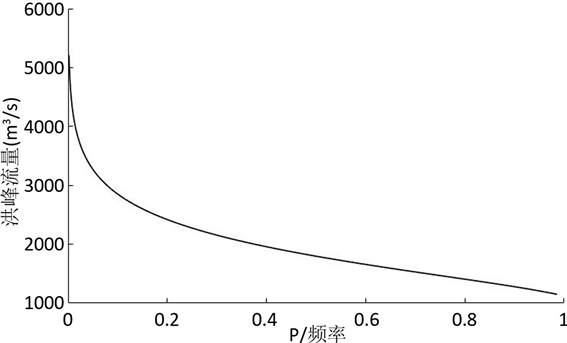Figure 5. Combined flood distribution curve

5. 结论

Combined Flood Research Based on Three-Dimensional Copula Function[J]. 水资源研究, 2020, 09(01): 33-41. https://doi.org/10.12677/JWRR.2020.91004

1. 1. KRSTANOVIC, P. F., SINGH, V. P. A multivariate stochastic flood analysis using entropy. In SINGH, V. P. (Ed.). Hydrologic frequency modeling. Dordrecht: Reidel, 1987: 515-539. https://doi.org/10.1007/978-94-009-3953-0_37

2. 2. CORREIA, F. N. Multivariate partial duration series in flood risk analysis. In SINGH, V. P. (Ed.). Hydrologic frequency modelling. Dordrecht: Reidel, 1987: 541-554. https://doi.org/10.1007/978-94-009-3953-0_38

3. 3. YUE, S. The bivariate lognormal distribution for describing joint statistical properties of a multivariate storm event. Environmetrics, 2002, 13: 811-819. https://doi.org/10.1002/env.483

4. 4. YUE, S. The gumbel logistic model for representing a multivariate storm event. Advances in Water Resource, 2001, 24: 179-185. https://doi.org/10.1016/S0309-1708(00)00039-7

5. 5. 郭生练, 刘章君, 熊立华, 等. 设计洪水计算方法研究进展与评价[M]. 北京: 中国水利水电出版社, 2005. GUO Shenglian, LIU Zhangjun, XIONG Lihua, et al. Research progress and evaluation of design flood calculation methods. Beijing: China Water Conservancy and Hydropower Press, 2005. (in Chinese)

6. 6. 费永法. 一种计算洪水条件概率的方法[J]. 水文, 1989(1): 18-22. FEI Yongfa. A method for calculating the probability of flood conditions. Hydrology, 1989(1): 18-22. (in Chinese)

7. 7. 郭生练, 闫宝伟, 肖义, 等. Copula函数在多变量水文分析计算中的应用及研究进展[J]. 水文, 2008, 28(3): 1-2. GUO Shenglian, YAN Baowei, XIAO Yi, et al. Application and research progress of Copula function in multivariate hydrological analysis and calculation. Hydrology, 2008, 28(3): 1-2. (in Chinese)

8. 8. 熊立华, 郭生练. Copula联接函数在多变量水文频率分析中的应用[J]. 武汉大学学报, 2005, 38(6): 16-17. XIONG Lihua, GUO Shenglian. Application of Copulas to multivariate hydrological frequency analysis. Journal of Wuhan University, 2005, 38(6): 16-17. (in Chinese)

9. 9. 李天元, 郭生练, 罗启华, 等. 双参数Copula函数在洪水联合分布中的应用研究[J]. 水文, 2011, 31(5): 24-28. LI Tianyuan, GUO Shenglian, LUO Qihua, et al. Application research of two-parameter Copula function in joint distribution of flood. Hydrology, 2011, 31(5): 24-28. (in Chinese)

10. 10. 刘章君, 郭生练, 李天元, 等. 设计洪水地区组成的区间估计方法研究[J]. 水利学报, 2015, 46(5): 543-544. LIU Zhangjun, GUO Shenglian, LI Tianyuan, et al. Research on interval estimation method for designing flood area. Journal of Hydraulic Engineering, 2015, 46(5): 543-544. (in Chinese)

11. 11. 陈路, 郭生练, 张洪刚, 等. 长江上游干支流洪水遭遇分析[J]. 水科学进展, 2011, 22(3): 323-330. CHEN Lu, GUO Shenglian, ZHANG Honggang, et al. Analysis on flood encounters of main tributaries in the upper reaches of the Yangtze. Progress in Water Science, 22(3): 323-330. (in Chinese)

12. 12. 傅湘, 王丽萍, 纪昌明. 洪水遭遇组合下防洪区的洪灾风险率估算[J]. 水电能源科学, 1999, 17(4): 23-26. FU Xiang, WANG Liping and JI Changmin. Estimation of flood risk rate in flood control areas under flood encounters. Hydropower Energy Science, 1999, 17(4): 23-26. (in Chinese)

13. 13. 蔡振华, 王祥三, 李娜. 沮漳河下游洪水遭遇地区组成规律分析及计算[J]. 中国农村水利水电, 2005(4): 23-26. CAI Zhenhua, WANG Xiangsan and LI Na. Analysis and calculation of composition rules of flood vulnerable area at the lower reach of Zu River and Zang River. China Rural Water and Hydropower, 2005(4): 23-26. (in Chinese)